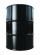# Container with water

The weight of a container with water is 2.48 kg. If the cast 75% water, the container with water has a weight of 0.98 kg. Determine the weight of the empty container. How much water was originally in the container?

Result

m =  0.48 kg
v =  2 kg

#### Solution:

m + v = 2.48
m+(1-75/100)*v = 0.98

m+v = 2.48
100m+25v = 98

m = 1225 = 0.48
v = 2

Calculated by our linear equations calculator.

Leave us a comment of example and its solution (i.e. if it is still somewhat unclear...):

Showing 0 comments:Be the first to comment!#### To solve this example are needed these knowledge from mathematics:

Our percentage calculator will help you quickly calculate various typical tasks with percentages. Do you have a system of equations and looking for calculator system of linear equations? Tip: Our volume units converter will help you with converion of volume units.

## Next similar examples:

1. Barrel with liquidBarrel with grain weight 297 kg. When it shed 48% of grain, ha weight 174 kg. What is the weight of empty barrel?
2. Linear systemSolve this linear system (two linear equations with two unknowns): x+y =36 19x+22y=720
3. WeightlifterWeightlifter lifted 75% of its weight. Determine how much weight lifted when he weighs 132 kg.
4. IronIron ore contains 57% iron. How much ore is needed to produce 20 tons of iron?
5. New refrigeratorNew refrigerator sells for 1024 USD, Monday will be 25% discount. How much USD will save, and what will be the price?
6. The ballThe ball was discounted by 10 percent and then again by 30 percent. How many percent of the original price is now?
7. Waiting roomIn the waiting room are people and flies. Together they have 15 heads and 50 legs (fly has 6 legs). How many people and flies are in the waiting room?
8. Seeds 2How many seeds germinated from 1000 pcs, when 23% no emergence?
9. Hotel roomsIn the 45 rooms, there were 169 guests, some rooms were three-bedrooms and some five-bedrooms. How many rooms were?
10. EnrollmentThe enrollment at a local college increased 4% over last year's enrollment of 8548. Find the increase in enrollment (x1) and the current enrollment (x2).
11. Percentages52 is what percent of 93?
12. Apples 2James has 13 apples. He has 30 percent more apples than Sam. How many apples has Sam?
13. Equations - simpleSolve system of linear equations: x-2y=6 3x+2y=4
14. Percents - easyHow many percent is 432 out of 434?
15. TVsProduction of television sets increased from 3,500 units to 4,200 units. Calculate the percentage of production increase.
16. EquationsSolve following system of equations: 6(x+7)+4(y-5)=12 2(x+y)-3(-2x+4y)=-44
17. CancerOf the 80 people 50 people ill cancer. What percentage of people isn't ill?